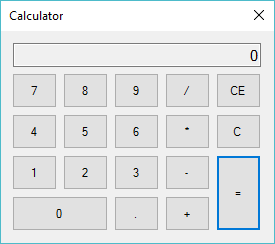# Windows Forms: How to make a Calculator in C#

## This post shows you how to create a simple calculator in c# windows application.

Dragging the TextBox, Button from the visual studio toolbox to your winform, then design a simple calculator as shown below.Next, Add code to handle your calculator form as the following c# code.

```double value;
string coperator;
bool check;```

Create the PNumber method allows you to display the numeric value that you enter in the TextBox control.

```//Handle number button
private void PNumber(object sender, EventArgs e)
{
if ((coperator == "+") || (coperator == "-") || (coperator == "*") || (coperator == "/"))
{
if (check)
{
check = false;
txtResult.Text = "0";
}
}
Button b = sender as Button;
if (txtResult.Text == "0")
txtResult.Text = b.Text;
else
txtResult.Text += b.Text;
}```

Create the POperator method allows you to convert text to numeric.

```//Handle operator button
private void POperator(object sender, EventArgs e)
{
Button b = sender as Button;
//Convert text to number
value = double.Parse(txtResult.Text);
coperator = b.Text;
txtResult.Text += b.Text;
check = true;
}```

Add the button click event handler to the Equal button allows you to calculate the result value.

```private void button20_Click(object sender, EventArgs e)
{
try
{
switch (coperator)
{
case "+":
txtResult.Text = (value + double.Parse(txtResult.Text)).ToString();
break;
case "-":
txtResult.Text = (value - double.Parse(txtResult.Text)).ToString();
break;
case "*":
txtResult.Text = (value * double.Parse(txtResult.Text)).ToString();
break;
case "/":
txtResult.Text = (value / double.Parse(txtResult.Text)).ToString();
break;
}
}
catch (Exception ex)
{
MessageBox.Show(ex.Message, "Message", MessageBoxButtons.OK, MessageBoxIcon.Error);
}
}```

Add the button click event handler to the C button allows you to reset the result value to zero.

```private void button5_Click(object sender, EventArgs e)
{
txtResult.Text = "0";
}```

Add the button click event handler to the CE button allows you to clear memory and reset the result value to zero.

```//Clear memory
private void button10_Click(object sender, EventArgs e)
{
txtResult.Text = "0";
value = 0;
}```

This is only a simple calculator that helps you perform some calculations. The application built is designed to emulate the windows calculator and is done in c# .net

VIDEO TUTORIAL